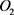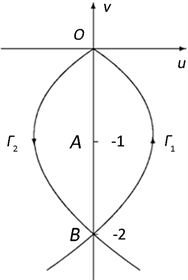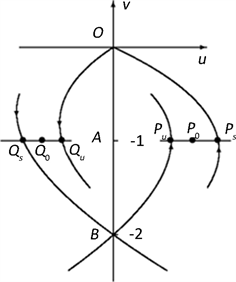﻿ 二维三次多项式系统的奇点与异宿环分支分析

二维三次多项式系统的奇点与异宿环分支分析The Analysis of Singularities and Bifurcation of Heteroclinic Loops of Two-Dimensional Cubic Polynomial Systems

Abstract: In this paper, the authors studied the problem of limit cycle bifurcated from heteroclinic loop for the cubic polynomial systemAssume that the heteroclinic orbit of the undisturbed system is broken after being disturbed. By analyzing the relative distances between the stable manifolds and the unstable manifolds of the disturbed system under small perturbations, combining the analysis of the types and stability of singularities, the authors obtained the conditions for the existence of stable and unstable limit cycle for the system.

1. 引言

2. 预备知识

$\left\{\begin{array}{l}\stackrel{˙}{x}=X\left(x,y\right)\\ \stackrel{˙}{y}=Y\left(x,y\right)\end{array}$ (2.1)

2.1. 环域定理  

2.2. Melnikov函数  

$\left\{\begin{array}{l}\stackrel{˙}{x}=f\left(x,y\right)\\ \stackrel{˙}{y}=g\left(x,y\right)\end{array}$ (2.2)

$\left\{\begin{array}{l}\stackrel{˙}{x}=f\left(x,y\right)+p{f}_{0}\left(x,y,p,q\right)\\ \stackrel{˙}{y}=g\left(x,y\right)+p{g}_{0}\left(x,y,p,q\right)\end{array}$ (2.3)

1) 系统(2.2)存在异宿于鞍点 ${O}_{1}$的两条异宿轨 ${\Gamma }_{1}$${\Gamma }_{2}$ 构成的异宿环 $\Gamma$${P}_{0}$${\Gamma }_{i}$$i=1,2$ 上任意一点，过 ${P}_{0}$ 作(2.2)的横截线l与 ${\Gamma }_{i}$${P}_{0}$ 点的外法线方向 $n$ 共线。

2) 扰动系统(2.3)在 ${O}_{1}$${O}_{2}$ 点附近的鞍点分别为 ${\stackrel{¯}{O}}_{1}$${\stackrel{¯}{O}}_{2}$ ，过 ${\stackrel{¯}{O}}_{i}$$i=1,2$ 的稳定流形 ${W}_{{\stackrel{¯}{O}}_{i}}^{s}$ 和不稳定流形 ${W}_{{\stackrel{¯}{O}}_{i}}^{u}$ 与l的交点分别为 ${P}_{i}^{s}$${P}_{i}^{u}$

$d\left({P}_{i}{}^{s},{P}_{i-1}^{u}\right)=\frac{pM}{\sqrt{{f}^{2}\left({P}_{0}\right)+{g}^{2}\left({P}_{0}\right)}}+o\left(p\right)$ (2.4)

3. 主要结果

$\left\{\begin{array}{l}\stackrel{˙}{x}=-y+\delta x+mxy+l{x}^{2}+{y}^{3}\\ \stackrel{˙}{y}=x\left(1+ax+by\right)\end{array}$ (3.1)

$p=\sqrt{{\delta }^{2}+{m}^{2}}$$q=\frac{\delta }{p}$$l=pk$$a=pr$$b=ps$ ，则 $p>0$$\delta =pq$$\delta$ 与q同号， $m=sign\left(m\right)p\sqrt{1-{q}^{2}}$ 。从而系统(3.1)化为：

$\left\{\begin{array}{l}\stackrel{˙}{x}=-y+{y}^{3}+p\left[qx+sign\left(m\right)\sqrt{1-{q}^{2}}xy+k{x}^{2}\right]\\ \stackrel{˙}{y}=x+p\left(r{x}^{2}+sxy\right)\end{array}$ (3.2)

$\left\{\begin{array}{l}x=u\\ y=v+1\end{array}$ ，则系统(3.2)化为：(3.3)

$\left\{\begin{array}{l}\stackrel{˙}{u}={v}^{3}+3{v}^{2}+2v\\ \stackrel{˙}{v}=u\end{array}$ (3.4)Figure 1. Heteroclinic Loop

$H\left(u,v\right)=\frac{1}{2}{u}^{2}-\frac{1}{4}{v}^{4}-{v}^{3}-{v}^{2}=h$ (3.5)

$A\left(0,-1\right)$ 代入(3.5)得 $H\left(0,-1\right)=-\frac{1}{4}$ 。经分析可知，当 $-\frac{1}{4} 时，(3.5)为(3.4)的异宿环 $\Gamma$ 内的一簇包围奇点 $A\left(0,-1\right)$ 的闭轨，此亦说明 $A\left(0,-1\right)$ 为(3.4)的中心。

$f={v}^{3}+3{v}^{2}+2v$$g=u$${f}_{0}=\left[q+sign\left(m\right)\sqrt{1-{q}^{2}}\left(v+1\right)+ku\right]u$${g}_{0}=\left[ru+s\left(v+1\right)\right]u$

${\Gamma }_{1}$ 上一点 ${P}_{0}\left(\frac{\sqrt{2}}{2},-1\right)$ ，不妨设此时 $t=0$ ，即 $u\left(0\right)=\frac{\sqrt{2}}{2}$$v\left(0\right)=-1$ ，过 ${P}_{0}\left(\frac{\sqrt{2}}{2},-1\right)$${\Gamma }_{1}$ 的截线 ${l}_{1}$

${n}_{1}$ ( ${\Gamma }_{1}$${P}_{0}$ 点的外法线方向)同向。设 ${W}_{O}^{s}$${W}_{B}^{u}$ 分别为 ${\Gamma }_{1}$ 经扰动破裂以后系统(3.3)过点 $O\left(0,0\right)$ 的稳定流形与过点 $B\left(0,-2\right)$ 的不稳定流形，且 ${W}_{O}^{s}$${W}_{B}^{u}$${l}_{1}$ 交点分别是 ${P}_{s}$${P}_{u}$ ，由预备知识2.2中的Melnikov函数知，在扰动充分小的情况下， ${P}_{s}$${P}_{u}$ 点的有向距离为：

$d\left({P}_{s},{P}_{u}\right)=\frac{p{M}_{1}}{\sqrt{{f}^{2}\left({P}_{0}\right)+{g}^{2}\left({P}_{0}\right)}}+o\left(p\right)$ (3.6)

${\Gamma }_{2}$ 的截线 ${l}_{2}$${n}_{2}$ ( ${\Gamma }_{2}$${Q}_{0}$ 点的外法线方向)同向。设 ${W}_{B}^{s}$${W}_{O}^{u}$ 分别为 ${\Gamma }_{2}$ 经扰动破裂以后系统(3.3)过点 $B\left(0,-2\right)$ 的稳定流形与过点 $O\left(0,0\right)$ 的不稳定流形(如图2所示)，且 ${W}_{B}^{s}$${W}_{O}^{u}$${l}_{2}$ 交点分别是 ${Q}_{s}$${Q}_{u}$ ，由预备知识2.2中的Melnikov函数知，在扰动充分小的情况下， ${Q}_{s}$${Q}_{u}$ 点的有向距离为：Figure 2. Relative position of orbits

$d\left({Q}_{s},{Q}_{u}\right)=\frac{p{M}_{2}}{\sqrt{{f}^{2}\left({Q}_{0}\right)+{g}^{2}\left({Q}_{0}\right)}}+o\left(p\right)$ (3.7)

$\begin{array}{c}{M}_{1}={\int }_{-\infty }^{+\infty }\left\{\left({v}^{3}+3{v}^{2}+2v\right)\left[ru+s\left(v+1\right)\right]-\left[q+sign\left(m\right)\sqrt{1-{q}^{2}}\left(v+1\right)+ku\right]u\right\}u\text{d}t\\ ={\int }_{-2}^{0}\left[-\frac{\sqrt{2}}{2}r\left({v}^{5}+5{v}^{4}+8{v}^{3}+4{v}^{2}\right)+s\left({v}^{4}+4{v}^{3}+5{v}^{2}+2v\right)\right]\text{d}v\\ \text{\hspace{0.17em}}\text{\hspace{0.17em}}+\frac{\sqrt{2}}{2}{\int }_{-2}^{0}\left[q\left({v}^{2}+2v\right)+sign\left(m\right)\sqrt{1-{q}^{2}}\left({v}^{3}+3{v}^{2}+2v\right)-\frac{\sqrt{2}}{2}k\left({v}^{4}+4{v}^{3}+4{v}^{2}\right)\right]\text{d}v\\ =-\frac{4}{15}s-\frac{8}{15}k-\frac{2\sqrt{2}}{3}q\end{array}$

$f={v}^{3}+3{v}^{2}+2v$$g=u$${P}_{0}\left(\frac{\sqrt{2}}{2},-1\right)$$f\left({P}_{0}\right)=0$$g\left({P}_{0}\right)=\frac{\sqrt{2}}{2}$ ，故

$d\left({P}_{s},{P}_{u}\right)=\frac{p{M}_{1}}{\sqrt{{f}^{2}\left({P}_{0}\right)+{g}^{2}\left({P}_{0}\right)}}+o\left(p\right)=\sqrt{2}p\left(-\frac{4}{15}s-\frac{8}{15}k-\frac{2\sqrt{2}}{3}q\right)+o\left(p\right)$

$\begin{array}{c}{M}_{2}={\int }_{-\infty }^{+\infty }\left\{\left({v}^{3}+3{v}^{2}+2v\right)\left[ru+s\left(v+1\right)\right]-\left[q+sign\left(m\right)\sqrt{1-{q}^{2}}\left(v+1\right)+ku\right]u\right\}u\text{d}t\\ ={\int }_{0}^{-2}\left[\frac{\sqrt{2}}{2}r\left({v}^{5}+5{v}^{4}+8{v}^{3}+4{v}^{2}\right)+s\left({v}^{4}+4{v}^{3}+5{v}^{2}+2v\right)\right]\text{d}v\\ \text{\hspace{0.17em}}\text{\hspace{0.17em}}-\frac{\sqrt{2}}{2}{\int }_{0}^{-2}\left[q\left({v}^{2}+2v\right)+sign\left(m\right)\sqrt{1-{q}^{2}}\left({v}^{3}+3{v}^{2}+2v\right)+\frac{\sqrt{2}}{2}k\left({v}^{4}+4{v}^{3}+4{v}^{2}\right)\right]\text{d}v\\ =\frac{4}{15}s+\frac{8}{15}k-\frac{2\sqrt{2}}{3}q\end{array}$

$f={v}^{3}+3{v}^{2}+2v$$g=u$${Q}_{0}\left(-\frac{\sqrt{2}}{2},-1\right)$$f\left({Q}_{0}\right)=0$$g\left({Q}_{0}\right)=-\frac{\sqrt{2}}{2}$ ，故

$d\left({Q}_{s},{Q}_{u}\right)=\frac{p{M}_{2}}{\sqrt{{f}^{2}\left({Q}_{0}\right)+{g}^{2}\left({Q}_{0}\right)}}+o\left(p\right)=\sqrt{2}p\left(\frac{4}{15}s+\frac{8}{15}k-\frac{2\sqrt{2}}{3}q\right)+o\left(p\right)$

2) 当 $q<0$ (即 $\delta <0$ )且 $\frac{4}{15}s+\frac{8}{15}k-\frac{2\sqrt{2}}{3}q>0$$-\frac{4}{15}s-\frac{8}{15}k-\frac{2\sqrt{2}}{3}q>0$ 时，系统(3.3)在 $\Gamma$ 的邻域内至少存在一条围绕 $A\left(0,-1\right)$ 的不稳定极限环。

NOTES

*通讯作者。

 叶彦谦. 极限环论[M]. 上海: 上海科技出版社, 1995.

 叶彦谦. 多项式微分系统定性理论[M]. 上海: 上海科技出版社, 1995.

 Jin, Y. (2001) The Problems of Homoclinic Bifurcation for Type (I) Planar Quadratic Polynomial Systems. Journal of Changde Teachers University (Natural Science Edition), 13, 13-15.

 郑庆玉. 二次微分系统(II)类方程的分支问题[J]. 曲阜师范大学学报, 2002, 28(3): 27-30.

 李光芹. 一类平面二次方程的极限环存在性[J]. 曲阜师范大学学报, 2002, 28(4): 53-55.

 金银来, 庄维欣. 二次微分系统(III)类方程的分支问题[J]. 常德师范学院学报(自然科学版), 2002, 14(4): 3-5.

 金银来. 一类Duffing方程的分支问题[J]. 吉首大学学报, 2001, 22(2): 50-53.

 程福德. 软弹簧型方程在摄动下分支出极限环[J]. 应用数学和力学, 1998(2): 121-125.

 东北师范大学微分方程教研室. 常微分方程[M]. 北京: 高等教育出版社, 2005.

 张芷芬, 丁同仁, 黄文灶, 董镇喜. 微分方程定性理论[M]. 北京: 科学出版社, 1985.

 马知恩, 周义仓. 常微分方程定性与稳定性方法[M]. 北京: 科学出版社, 2008.

 Luo, D., Wang, X., Zhu, D. and Han, M. (1997) Bifurcation Theory and Methods of Dynamical Systems. In: Advanced Series in Dynamical Systems, World Scientific, Singapore.
https://doi.org/10.1142/2598

Top